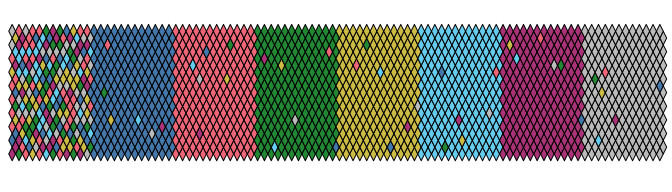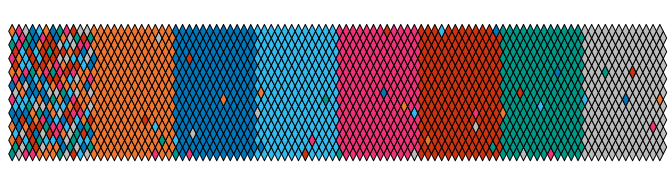# Paul Tol’s Colour Schemes

#### 2022-06-18

``library(khroma)``

## Introduction

Tol (2021) offers carefully chosen schemes, ready for each type of data, with colours that are:

• Distinct for all people, including colour-blind readers,
• Distinct from black and white,
• Distinct on screen and paper,
• Matching well together.

All the scales presented in Paul Tol’s technical note (issue 3.2, 2021-08-18) are implemented here, for use with base R graphics or ggplot2.

## Qualitative data

According to Paul Tol’s technical note, the bright, contrast, vibrant and muted colour schemes are colour-blind safe.

The light colour scheme is reasonably distinct for both normal or colour-blind vision and is intended to fill labelled cells.

The pale and dark schemes are not very distinct in either normal or colour-blind vision and should be used as a text background or to highlight a cell in a table.

The qualitative colour schemes must be used as given (no interpolation): colours are picked up to the maximum number of supported values. Refer to the original document for details about the recommended uses (see references).

Scheme Max. colours
`bright` 7
`high contrast` 3
`vibrant` 7
`muted` 9
`medium contrast` 3
`pale` 6
`dark` 6
`light` 9

### bright

``````bright <- colour("bright")
plot_scheme(bright(7), colours = TRUE, names = TRUE, size = 0.9)``````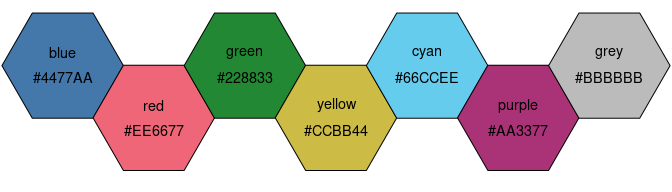### high contrast

``````highcontrast <- colour("high contrast")
plot_scheme(highcontrast(3), colours = TRUE, names = TRUE, size = 0.9)``````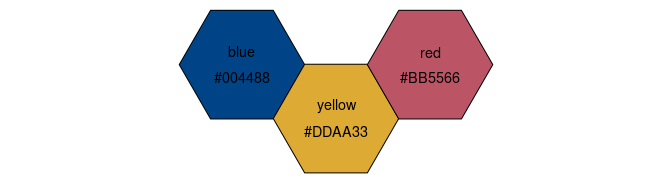### vibrant

``````vibrant <- colour("vibrant")
plot_scheme(vibrant(7), colours = TRUE, names = TRUE, size = 0.9)``````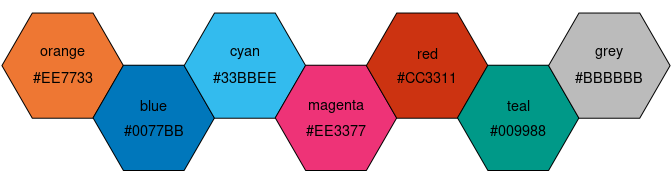### muted

``````muted <- colour("muted")
plot_scheme(muted(9), colours = TRUE, names = TRUE, size = 0.9)``````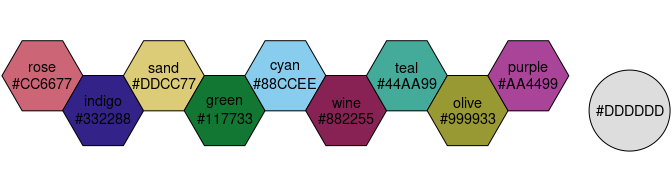### medium contrast

``````mediumcontrast <- colour("medium contrast")
plot_scheme(mediumcontrast(6), colours = TRUE, names = TRUE, size = 0.9)``````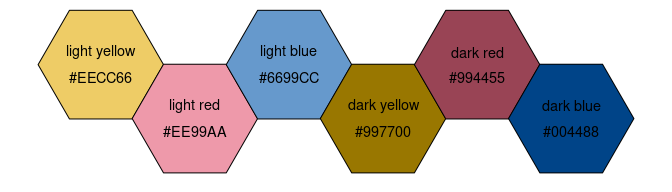### pale and dark

``````pale <- colour("pale")
plot_scheme(pale(6), colours = TRUE, names = TRUE, size = 0.9)``````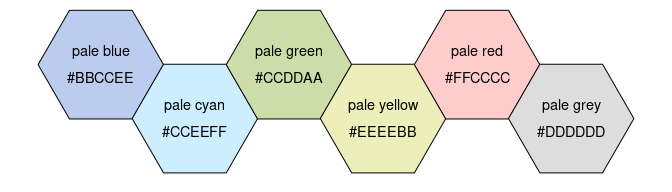``````
dark <- colour("dark")
plot_scheme(dark(6), colours = TRUE, names = TRUE, size = 0.9)``````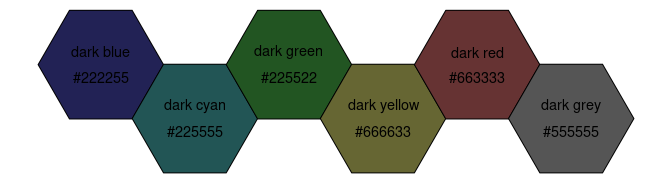### light

``````light <- colour("light")
plot_scheme(light(9), colours = TRUE, names = TRUE, size = 0.9)``````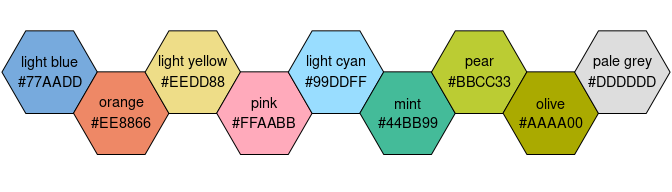## Diverging data

If more colours than defined are needed from a given scheme, the colour coordinates are linearly interpolated to provide a continuous version of the scheme.

Scheme Num. of colours Bad data
`sunset` 11 #FFFFFF
`BuRd` 9 #FFEE99
`PRGn` 9 #FFEE99

### sunset

``````sunset <- colour("sunset")
plot_scheme(sunset(9), colours = TRUE, size = 0.9)``````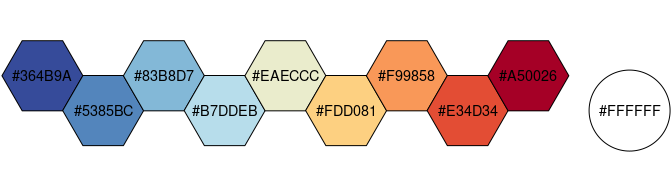### BuRd

``````BuRd <- colour("BuRd")
plot_scheme(BuRd(9), colours = TRUE, size = 0.9)``````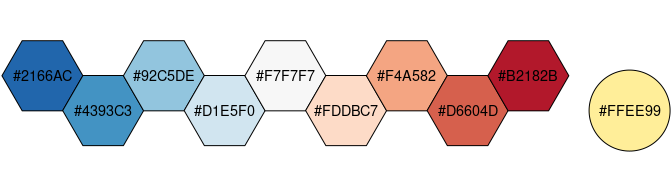### PRGn

``````PRGn <- colour("PRGn")
plot_scheme(PRGn(9), colours = TRUE, size = 0.9)``````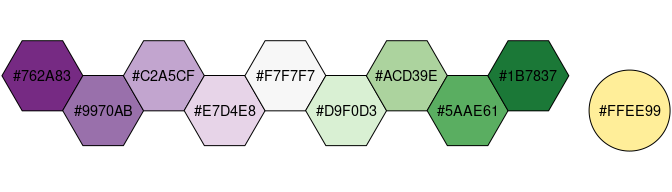## Sequential data

If more colours than defined are needed from a given scheme, the colour coordinates are linearly interpolated to provide a continuous version of the scheme, with the exception of the discrete rainbow scheme (see below).

Scheme Num. of colours Bad data
`YlOrBr` 9 #888888
`iridescent` 23 #999999
`discrete rainbow` 23 #777777
`smooth rainbow` 34 #666666

### YlOrBr

``````YlOrBr <- colour("YlOrBr")
plot_scheme(YlOrBr(9), colours = TRUE, size = 0.9)``````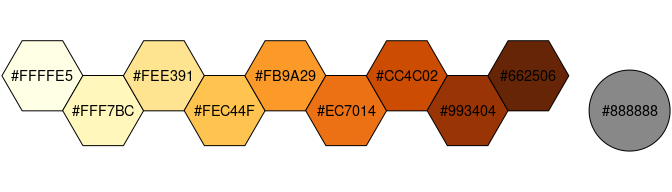### iridescent

``````iridescent <- colour("iridescent")
plot_scheme(iridescent(23), colours = TRUE, size = 0.5)``````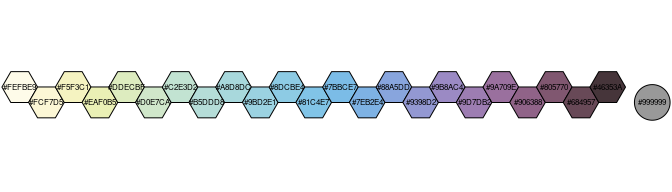### rainbow

As a general rule, ordered data should not be represented using a rainbow scheme. There are three main arguments against such use (Tol 2021):

• The spectral order of visible light carries no inherent magnitude message.
• Some bands of almost constant hue with sharp transitions between them, can be perceived as jumps in the data.
• Colour-blind people have difficulty distinguishing some colours of the rainbow.

If such use cannot be avoided, Paul Tol’s technical note provides two colour schemes that are reasonably clear in colour-blind vision. To remain colour-blind safe, these two schemes must comply with the following conditions:

discrete rainbow
This scheme must not be interpolated.
smooth rainbow
This scheme does not have to be used over the full range (Tol 2021 suggests starting at purple).
``````discrete_rainbow <- colour("discrete rainbow")
plot_scheme(discrete_rainbow(14), colours = TRUE, size = 0.7)``````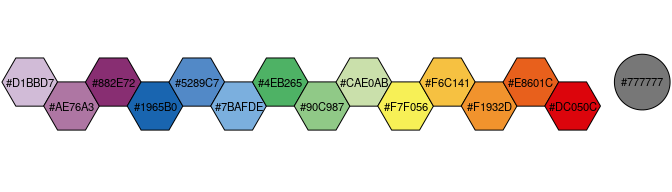When using the smooth rainbow scheme:

• Start off-white instead of purple if the lowest data value occurs often;
• End at red instead of brown the highest data value occurs often.
``````smooth_rainbow <- colour("smooth rainbow")

## Start at purple instead of off-white
plot(smooth_rainbow(256, range = c(0.25, 1)))````````````## End at red instead of brown
plot(smooth_rainbow(256, range = c(0, 0.9)))``````## Diagnostic maps

### Qualitative colour schemes